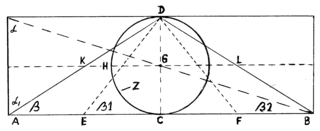## Sunday, October 13, 2013

### PRECESSION OF THE EQUINOX

The base length of the Great Pyramid: 23,192.867 cm

The Pyramid’s Code: 1 day = 2.466586266 cm

1000 x 23,192.867 cm = 23,192,867 cm

23,192,867 cm = 9,402,820.132 days = 25,744.08237 years =  circular movement of the Earth's axis or precession of the equinoxes (annual motion is about  0.013983796° =  50.3416661 seconds of arc per year, or 1 degree every 71.5113407 years.

## Friday, October 4, 2013

### Geometrical scheme of the UniverseGeometrical scheme of the Universe
.
DC = diameter of the circle Z =
GD = radius of the circle Z =
Angle β1 = Pyramid’s ascent = 51,85399754°
Tangent β1 = 1,273240621
CircumferenceC = (d/tang. β1) x 4
AreaA = (r²/tang. β1) x 4
Angle β = ascent of the King’s Chamber north channel = 32,48165854°
Tangent β = 0,63662031
Circumference(d/tang. β) x 2
Area
(r²/tang. β) x 2
Angle β2 = 17,65680115°
Tangent β2 = 0,318310155 = 1 : 3,14159
CircumferenceC = d/tang. β2 = AB
AreaA = r²/tang. β2

DH = r/tangβ KG = GL = AE EC = CF = FB = 1/4 AB
DC = D/tang. β = AC = CB = 1/2 AB

Tangent of the angle α (Alpha) = 3,14159 = Pi
Tangent of the angle Alpha= 1,570795 = Pi/2

The rectangle’s Area = Area of the sphere around the circle Z